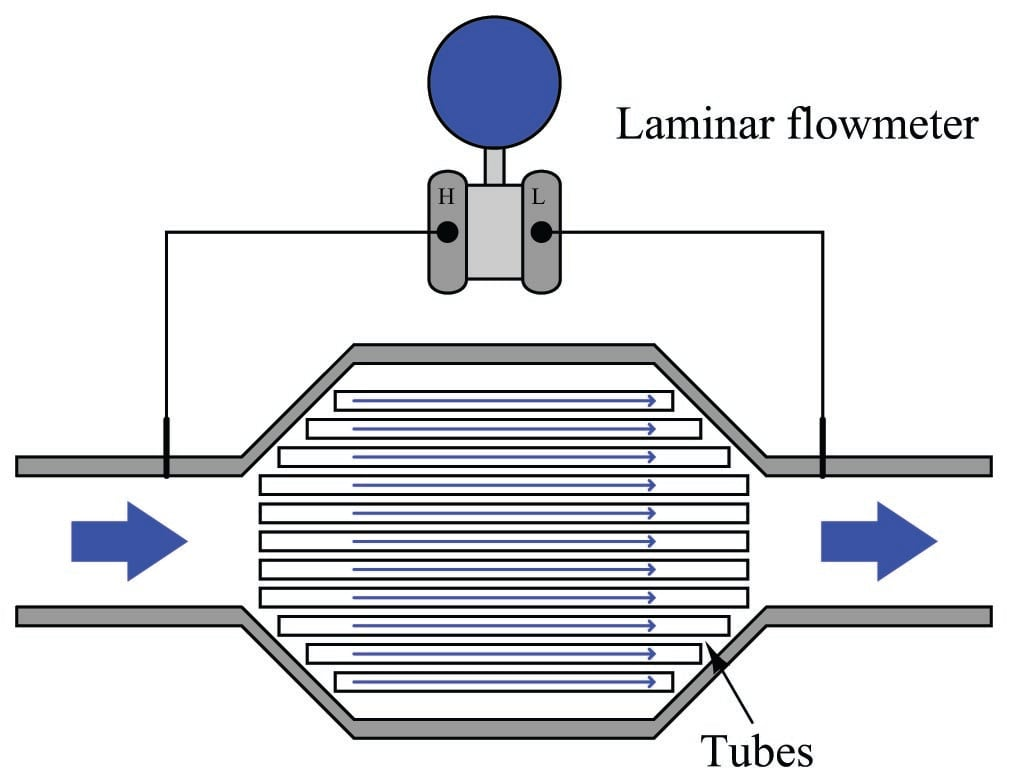# Laminar Flowmeters

## Chapter 22 - Introduction to Continuous Fluid Flow Measurement

A unique form of differential pressure-based flow measurement deserves its own section in this flow measurement chapter, and that is the laminar flowmeter.

Laminar flow is a condition of fluid motion where viscous (internal fluid friction) forces greatly overshadow inertial (kinetic) forces. A flowstream in a state of laminar flow exhibits no turbulence, with each fluid molecule traveling in its own path, with limited mixing and collisions with adjacent molecules. The dominant mechanism for resistance to fluid motion in a laminar flow regime is friction with the pipe or tube walls. Laminar flow is qualitatively predicted by low values of Reynolds number.

This pressure drop created by fluid friction in a laminar flowstream is quantifiable, and is expressed in the Hagen-Poiseuille equation:

$Q = k \left({{\Delta P D^4} \over {\mu L}}\right)$

Where,

$$Q$$ = Flow rate

$$\Delta P$$ = Pressure dropped across a length of pipe

$$D$$ = Pipe diameter

$$\mu$$ = Fluid viscosity

$$L$$ = Pipe length

$$k$$ = Coefficient accounting for units of measurement

Laminar flowmeter elements generally consist of one or more tubes whose length greatly exceeds the inside diameter, arranged in such a way as to produce a slow-moving flow velocity. An example is shown here:The expanded diameter of the flow element ensures a lower fluid velocity than in the pipes entering and exiting the element. This decreases the Reynolds number to the point where the flow regime exhibits laminar behavior. The large number of small-diameter tubes packed in the wide area of the element provide adequate wall surface area for the fluid’s viscosity to act upon, creating an overall pressure drop from inlet to outlet which is measured by the differential pressure transmitter. This pressure drop is permanent (no recovery of pressure downstream) because the mechanism of pressure drop is friction: total dissipation (loss) of energy in the form of heat.

Another common form of laminar flow element is simply a coiled capillary tube: a long tube with a very small inside diameter. The small inside diameter of such a tube makes wall-boundary effects dominant, such that the flow regime will remain laminar over a wide range of flow rates. The extremely restrictive nature of a capillary tube, of course, limits the use of such flow elements to very low flow rates such as those encountered in the sampling networks of certain analytical instruments.

A unique advantage of the laminar flowmeter is its linear relationship between flow rate and developed pressure drop. It is the only pressure-based flow measurement device for filled pipes that exhibits a linear pressure/flow relationship. This means no “square-root” characterization is necessary to obtain linear flow measurements with a laminar flowmeter. The big disadvantage of this meter type is its dependence on fluid viscosity, which in turn is strongly influenced by fluid temperature. Thus, all laminar flowmeters require temperature compensation in order to derive accurate measurements, and some even use temperature control systems to force the fluid’s temperature to be constant as it moves through the element.

Laminar flow elements find their widest application inside pneumatic instruments, where a linear pressure/flow relationship is highly advantageous (behaving like a “resistor” for instrument air flow) and the viscosity of the fluid (instrument air) is relatively constant. Pneumatic controllers, for instance, use laminar restrictors as part of the derivative and integral calculation modules, the combination of “resistance” from the restrictor and “capacitance” from volume chambers forming a sort of pneumatic time-constant ($$\tau$$) network.

Published under the terms and conditions of the Creative Commons Attribution 4.0 International Public License